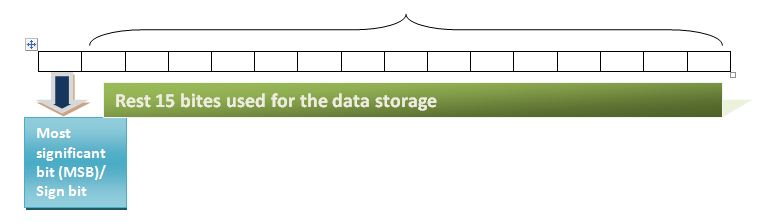# Explore hunderds of solved example topic wise.

## Data types

### Data types in C language

#### What is Data type?

As you have seen in the previous article, a variable is declared as given below...

int a; //variable declaration

1. In the above program code snippet, int is called a data type and a is a variable of that data type.
2. Data type describes the content a variable can store.
3. Data type also explains how data will be stored (the organization) in memory.

Data types can be classified into two broad categories...

1. Primitive (Basic) data type
2. Composite (Secondary) data types / User-defined data types

In this article we will only discuss primitive data types, User-defined data types will be discussed later.

#### Primitive data types

Data types which are defined by the system/programming language are called primitive data type. These data type defines a single entity, which can't be divided further.
List of primitive data types along with their memory occupied and the allowed range are described as below...
1. Integer Data types
2. Real Data types
3. Character Datatypes

#### Integral data types

Data typesKeyword usedMemory OccupiedAllowed Range
Short Integersshort / short int / int2 bytes-32768 to 32767
Long integerslong/long int4 bytes-2147483648 to 2147483647
1. Short Integers can be defined by using keywords short/short int/int.
2. Most of the c compilers treat int data type equivalent to short but it is not true for all compilers. Some compilers treat it equivalent to long. But in any compiler, the thing which is common is as follows.
3. short <= int <= long

#### Real data types

Data typesKeyword usedMemory OccupiedAllowed Range
floatfloat4 bytes (6 digits precision)3.4e-38 to 3.4e38
doubledouble(14 digits precision)8 bytes1.7e-308 to 1.7e+308
long doublelong double(14 digits precision)10 bytes3.4e-4932 to 1.1e+4932

#### Character data types

Data typesKeyword usedMemory OccupiedAllowed Range
characterchar1 byte-128 to 127

#### Range Calculation

As shown above, the range of each integral data type is shown... How to calculate this range? This article demonstrates you...1. An integer occupies 2 bytes (16 bits) in memory and the memory map of the integer data type is shown in the above figure.
2. As shown in the above figure the leftmost bit of integer is called MSB or sign bit, which stores the sign of the number.
3. As we know in computers memory everything is stored in the form of 0 and 1.
4. So in the sign bit,1 means -ve number and 0 means +ve numbers.
5. Rest of the 15 bits are used to store data and there can 215 combinations are possible to organize in 15 places. so the range maximum numbers that can be made in this memory are 215.
6. These 215 combinations are made with a +ve sign and -ve sign, and the range will be -215 to 215 - 1 would result -32768 to 32767.
7. So the general formula to calculate rang is as given.

-2 (n-1) to 2 (n-1) - 1 where n is no. of bits occupied.

#### unsigned keyword

1. We know that integer occupies 2 bytes(16 bits) among them 1 bit is used for storing the sign.
2. If the number is positive and we don't want to sacrifice the sign bit to store the sign, then the variable declaration should be prefixed with the unsigned keyword.
3. The benefit of creating a variable unsigned is that the range will be double.
Data TypeRange
unsigned int a;0 to 65535 (0 to 216-1)
unsigned char a;0 to 65535 (0 to 28-1)